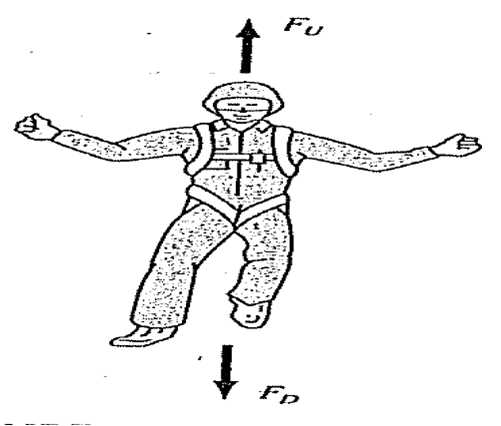help: euler() prg on home mode
05-30-2017, 02:36 PM (This post was last modified: 06-07-2017 02:59 PM by compsystems.)
Post: #1compsystemsSenior Member Posts: 1,342 Joined: Dec 2013
help: euler() prg on home mode
Hello, the next numerical code, I want to run it in HOME MODE.

The definition v(t) does not allow me to define it within the code, Why?

PHP Code:
// file euler.hppprgmexport euler()BEGIN    local g,c,m,t;    local vi,ti,vf,tf,iteration;    //local v;    purge(g,c,m,t,vi,ti,vf,tf);    v(t):= vi+(g-(c/m)*vi)*(t-ti);    g:=9.81; c:=12.5; m:=68.1;    vi:=0;    ti:=0;    iteration:=1;    print; // clear terminal view (output)        print("euler");    print("press [↓] for next iteration" );    print("press [on] for exit" );    wait(1);       FOR tf FROM 0 TO 10 STEP 2 DO        print; // clear terminal view (output)        print("iteration = "+iteration);        vf:=v(tf);        print("vi = "+vi+" m/s");        print("ti = "+ti+" s");        print("tf = "+tf+" s");        print("vf("+tf+") = "+vf+" m/s");        print("");        vi:=vf;        ti:=tf;        iteration:=iteration+1;         wait();    END;    print("Iterations finished," );    return("Done");END;
Source: NUMERICAL METHODS FOR ENGINEERS Steven C. Chapra & Raymond P. CanaleI managed to run it by doing the following trick.
Icomment the line // v(t):= vi+(g-(c/m)*vi)*(t-ti);
and I put in the line of entry (CAS MODE) the expression
v(t):= vi + (g- (c / m) * vi) * (t-ti);

euler() returns [] =(
but
[shift](program editor) euler
[DEBUG][CONT] [enter] ...[enter] ... ok
The program shows me screen by screen what I want
vi=0 m/s, ti=0 s , tf=0 s
v(0) = 0 m/s

vi=0, ti=0, tf=2
v(2) = 19.62 m/s

vi=19.62 m/s, ti=2 s, tf=4 s
v(4) = 32.04 m/s

vi=32.04 m/s, ti=4 s, tf=6 s
v(6") = 39.90 m/s
...

Please someone can help me by rewriting the above code to work well

Thanks

PS: In CAS mode if it works fine but how can I clean each screen? The print; command does not seem to be working. I have to clean each screen because the terminal view does not progress after the first impression screen is completed, (even hp-prime is a single process) should allow split screen and more of a process as did the hp48,

PHP Code:
// file euler1.hppprgm// version simple MODO CAS#cas  euler1():=  BEGIN    local g,c,m,t;    local vi,ti,vf,tf,iteration;    local v;    purge(g,c,m,t,vi,ti,vf,tf);    v(t):= vi+(g-(c/m)*vi)*(t-ti);    g:=9.81; c:=12.5; m:=68.1;    vi:=0;    ti:=0;    iteration:=1;    PRINT(); // clear terminal view (output)    print("euler1");    print("press [↓] for next iteration" );    print("press [on] for exit" );    wait(1);          FOR tf FROM 0 TO 10 STEP 2 DO      PRINT(); // clear terminal view (output)      print("iteration = "+iteration);      vf:=v(tf);      print("vi = "+vi+" m/s");      print("ti = "+ti+" s");      print("tf = "+tf+" s");      print("vf("+tf+") = "+vf+" m/s");      print("");      vi:=vf;      ti:=tf;      iteration:=iteration+1;       wait();    END;    print("Iterations finished" );    return("Done");  END;#end

Debug mode does not work for CAS MODE = (, for this reason I want to create it in HOME MODE
05-30-2017, 03:59 PM (This post was last modified: 05-30-2017 04:13 PM by toml_12953.)
Post: #2
 toml_12953Senior Member Posts: 2,033 Joined: Dec 2013
RE: help: euler() prg on home mode
(05-30-2017 02:36 PM)compsystems Wrote:  Hello, the next numerical code, I want to run it in HOME MODE.

The definition v(t) does not allow me to define it within the code, Why?

PHP Code:
EXPORT euler()BEGIN    local g,c,m,t;    local vi,ti,vf,tf;        local v;    purge(g,c,m,t,vi,ti,vf,tf);    g:=9.81; c:=12.5; m:=68.1;    v(t):= 'vi+(g-(c/m)*vi)*(t-ti)';    vi:=0;  ti:=0;    //print();    FOR tf FROM 0 TO 60 STEP 2 DO        print(); // clear terminal view (output)        vf:=v(tf);        print("vi = "+vi);        print("ti = "+ti);        print("tf = "+tf);        print("vf("+tf+") = "+vf);        print("");        vi:=vf;        ti:=tf;        wait();    END;    return "Done";END;
Source: NUMERICAL METHODS FOR ENGINEERS Steven C. Chapra & Raymond P. Canale

euler (all lowercase) is a CAS function so I renamed it to EULER. Also, I'm not sure about your function definition. The following program works:

Code:
g;c;m;t; vi;ti;vf;tf; v(t) BEGIN     return vi+(g-(c/m)*vi)*(t-ti); END; EXPORT EULER() BEGIN     purge(g,c,m,t,vi,ti,vf,tf);     g:=9.81; c:=12.5; m:=68.1;     vi:=0; ti:=0;     print();     FOR tf FROM 0 TO 60 STEP 2 DO         print();         vf:=v(tf);         print("vi = "+vi);         print("ti = "+ti);         print("tf = "+tf);         print("vf("+tf+") = "+vf);         print("");         vi:=vf;         ti:=tf;         wait();     END;     return "Done"; END;

Tom L
Cui bono?
05-30-2017, 04:18 PM (This post was last modified: 06-07-2017 03:06 PM by compsystems.)
Post: #3compsystemsSenior Member Posts: 1,342 Joined: Dec 2013
RE: help: euler() prg on home mode
Thanks toml_12953, but the file euler1.hppprgm does not clean the terminal view why?

Version calling an external function v(t)
PHP Code:
// file euler2.hppprgm// version simple MODO HOME (NUM)local g;c;m;t;local vi;ti;vf;tf;iteration;local v(t)BEGIN    return vi+(g-(c/m)*vi)*(t-ti);END;export euler2()BEGIN  purge(g,c,m,t,vi,ti,vf,tf);  g:=9.81; c:=12.5; m:=68.1;  vi:=0;  ti:=0;  iteration:=1;  print; // clear terminal view (output)  print("press [↓] for next iteration" );  print("euler2");  print("press [on] for exit" );  wait(1);     FOR tf FROM 0 TO 10 STEP 2 DO    print; // clear terminal view (output)    print("iteration = "+iteration);    vf:=v(tf);    print("vi = "+vi+" m/s");    print("ti = "+ti+" s");    print("tf = "+tf+" s");    print("vf("+tf+") = "+vf+" m/s");    print("");    vi:=vf;    ti:=tf;    iteration:=iteration+1;     wait();  END;  print("Iterations finished" );  return("Done");END;

Some alternative way for file euler2.hppprgm but without call an external function v(t) and not #cas...end prgm, I think the CAS() command is required
05-30-2017, 05:03 PM
Post: #4
 toml_12953Senior Member Posts: 2,033 Joined: Dec 2013
RE: help: euler() prg on home mode
(05-30-2017 04:18 PM)compsystems Wrote:  Thanks toml_12953, but the file euler1.hppprgm does not clean the terminal view why?

Have you tried

print();

print; ?

Tom L

Tom L
Cui bono?
05-30-2017, 05:17 PM
Post: #5compsystemsSenior Member Posts: 1,342 Joined: Dec 2013
RE: help: euler() prg on home mode
Thanks again
The solution we do not remember
print() in CASS prgm prints NULL
PRINT() clears the terminal view

Please HP-prime team try to solve this problem with a specific catalog for each CAS/HOME mode and, in the program editor, please check that the program names can not be compiled if it is a reserved system word

Pending (home) version without subroutines of euler2.hppprgm

Thanks
 « Next Oldest | Next Newest »

User(s) browsing this thread: 1 Guest(s)